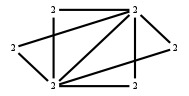# The Markov Bases Database

## G161g_bin

The binary graphical model of G161.

It is a hierarchical model of 6 variables. The dimension of the model is 19.The cardinality of the statespace is 64.

### Properties of the Markov basis

 Markov degree 2 220

 degree 2 220

The model has the following properties:

• All variables are binary.
• It is a graphical model.
• The semigroup is normal.### FILES

 Markov basis: sufficient statistics matrix: G161g_bin.mar (41.47 kb) G161g_bin.mat (4.04 kb) G161g_bin.mod (49 b) G161g_bin.tar.gz (41.66 kb)